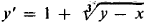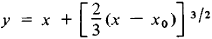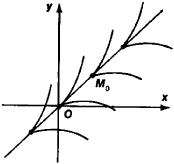# Singular Solution

Also found in: Wikipedia.

## singular solution

[′siŋ·gyə·lər sə′lü·shən]
(mathematics)
For a differential equation, a solution that is not generic, that is, not obtainable from the general solution. Also known as singular integral.
McGraw-Hill Dictionary of Scientific & Technical Terms, 6E, Copyright © 2003 by The McGraw-Hill Companies, Inc.
The following article is from The Great Soviet Encyclopedia (1979). It might be outdated or ideologically biased.

## Singular Solution

A singular solution of a differential equation is a solution at every point of which uniqueness is violated. For the equation y′ = f(x, y), this means that through each point of the singular solution there pass several different integral curves with the same tangent. When f(x, y) is continuous, this situation is possible only if a Lipschitz condition for y is not satisfied at the points of the singular solution for f(x, y). For example, the line y = x is a singular solution for the equation(see Figure 1); through any point M0 (x0, y0) of this line, in addition to the line itself, there pass the integral curvesGeometrically, the singular solution is the envelope of the family of integral curves Φ (x, y, C) = 0 that form the general integral of the equation.Figure 1

For the differential equation F(x, y, y′) = 0, the discriminant curve D(x, y) = 0 is defined as the result of the elimination of the parameter p = y′ from the system F(x, y, p) = 0 and F’p (x, y, p) = 0. Only part of this curve is, in general, a singular solution.

### REFERENCE

Stepanov, V. V. Kurs differentsial’nykh uravnenii, 8th ed. Moscow, 1959.